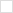This wiki is locked. Future workgroup activity and specification development must take place at our new wiki. For more information, see this blog post about the new governance model and this post about changes to the website.
TWiki>Main Web>PmHome>MetricsHome>MetricsWorkingDocuments>MetricsEMS10RestApi>MetricsEMS10RestApiDataModel>MetricsEmsCumulativeDistributionFunction (24 Sep 2010, ArthurRyman)

# ems:CumulativeDistributionFunction

## Description

Any probability distribution can be represented by its cumulative distribution function? . The cumulative distribution function for a given value gives the probability that an observation of the quantiy (random variable) will be be less than or equal to the given value. This is sometimes referred to as the confidence level or probability level of an estimate, e.g. a statement such as "the project will take 6 months to complete with a probability of 80%" means that there is a 0.80 probability that the observed duration of the project will be less than or equal to 6 months, or, equivalently, that the value of the cumulative distribution function at 6 months is 0.80.

The cumulative distribution function may vary continuously, however, it can be approximated by giving its value a finite set of sample points. Values between the sample points can be approximated using simple linear interpolation.

In general, a probability distribution may have non-zero values for arbitrarily small or large values, i.e. the cumulative probability may only reach 0 at negative infinity and 1 at positive infinity. However, for software estimates, the range of possible values is finite in practice and it is useful to include these low and high values in the representation of the cumulative distribution function.

An ems:CumulativeDistributionFunction resource represents a cumulative distribution function.

## Properties

Property Range Type Occurrence Edits Description
ems:low datatype xsd:double exactly-one read-write The low value.
ems:cdfPoint local inline resource ems:CdfPoint one-or-more read-write A cumulative distribution function data point.
ems:high datatype xsd:double exactly-one read-write The high value.

### ems:low

This required property is the low value of the distribution. The probability is 0 below this value and goes positive above it.

### ems:cdfPoint

This required, multi-valued property gives inline ems:CdfPoint resources which specify the cumulative probability of each sample point. Each sample value MUST be between the high and low. The cumulative probability of each sample point MUST increase as the value increases.

### ems:high

This required property is the high value of the distribution. The probability is 1 above this value and goes less than 1 below it.

## ems:CdfPoint

This inline resource represents a sample point of the cumulative distribution function. The point gives the cumulative probability for a given value of the random variable.

It has the following properties:

Property Range Type Occurrence Edits Description
ems:numericValue datatype xsd:double exactly-one read-write The value of the random variable.
ems:probability datatype xsd:double exactly-one read-write The cumulative probability.

### ems:numericValue

This required property is the value for which the cumulative probability of this quantile is attained, i.e. the probability that a measurement gives a result less than or equal to this value is equal to the cumulative probability of this quantile.

### ems:probability

This required property is the cumulative probability of this sample point.

## Examples

### Cumulative Distribution Function 1

Consider the following table of cumulative probabilities for duration of a project:

duration (months) cumulative probability
4 0.00
6 0.10
8 0.50
10 0.80
12 0.95
14 1.00

This cumulative distribution function has the following representation as an ems:CumulativeDistributionFunction resource:

###### Listing of Cumulative Distribution Function 1
```<?xml version="1.0"?>
<ems:CumulativeDistributionFunction
xmlns:ems="http://open-services.net/ns/ems#" xmlns:rdf="http://www.w3.org/1999/02/22-rdf-syntax-ns#">
<ems:low rdf:datatype="http://www.w3.org/2001/XMLSchema#double">4</ems:low>
<ems:cdfPoint>
<ems:CdfPoint>
<ems:numericValue rdf:datatype="http://www.w3.org/2001/XMLSchema#double">6</ems:numericValue>
<ems:probability rdf:datatype="http://www.w3.org/2001/XMLSchema#double">0.10</ems:probability>
</ems:CdfPoint>
</ems:cdfPoint>
<ems:cdfPoint>
<ems:CdfPoint>
<ems:numericValue rdf:datatype="http://www.w3.org/2001/XMLSchema#double">8</ems:numericValue>
<ems:probability rdf:datatype="http://www.w3.org/2001/XMLSchema#double">0.50</ems:probability>
</ems:CdfPoint>
</ems:cdfPoint>
<ems:cdfPoint>
<ems:CdfPoint>
<ems:numericValue rdf:datatype="http://www.w3.org/2001/XMLSchema#double">10</ems:numericValue>
<ems:probability rdf:datatype="http://www.w3.org/2001/XMLSchema#double">0.80</ems:probability>
</ems:CdfPoint>
</ems:cdfPoint>
<ems:cdfPoint>
<ems:CdfPoint>
<ems:numericValue rdf:datatype="http://www.w3.org/2001/XMLSchema#double">12</ems:numericValue>
<ems:probability rdf:datatype="http://www.w3.org/2001/XMLSchema#double">0.95</ems:probability>
</ems:CdfPoint>
</ems:cdfPoint>
<ems:high rdf:datatype="http://www.w3.org/2001/XMLSchema#double">14</ems:high>
</ems:CumulativeDistributionFunction>
```Български Cesky Dansk Deutsch English Español Français Italiano 日本語 Nederlands Polski Português Русский Svenska 简体中文 簡體中文